# Myristoylator - Documentation

N-terminal myristoylation is a post-translational modification that causes the addition of a myristate to a glycine in the N-terminal end of the amino acid chain. We used ensembles of neural networks, in order to learn to discriminate positive and negative sequences for myristoylation. Note that the neural network predictor has not been trained to predict myristoylation of internal glycines. The score S of our predictor is based on the average responses of 25 artificial neural networks. The next table illustrates an example of the output of the neural network predictor.

Positive Negative
Average response of 25 neural networks 0.988849 0.011067
Counters 25 0

Score = 0.988849 - 0.011067 = 0.977782 (High Confidence)

More precisely, the average of positive answers is 0.988849 and the average of negative responses is 0.011067 (average responses are between 0 and 1). The last row of the table gives the number of positive and negative responses among the 25 networks.

The score S is defined as: S = Positive - Negative. The reason is that under several hypothesis, neural network outputs converge towards the posterior probability of the class given the observations. A score close to one involves a high confidence for myristoylation, whereas a score close to -1 is a strong decision in favour of the absence of myristoylation.

For positive scores we defined:
• 0.0 < S < 0.4 ---> Low Confidence
• 0.4 < S < 0.85 --> Medium Confidence
• 0.85 < S < 1 ---> High Confidence
In the remaining paragraphs we describe how neural networks were created and trained to learn myristoylated and non-myristoylated proteins.

Artificial neural networks are able to learn concepts (also denoted as classes), by viewing many times representative examples. Basically, neural networks are several simple processors calculating weighted sum of inputs and activation functions (see next figure).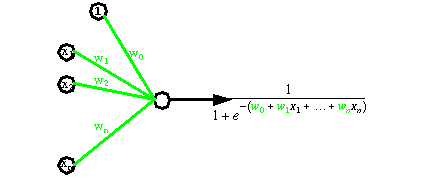Neural networks are able to classify objects such as tables and chairs from their weight and their height. See for instance the figure below.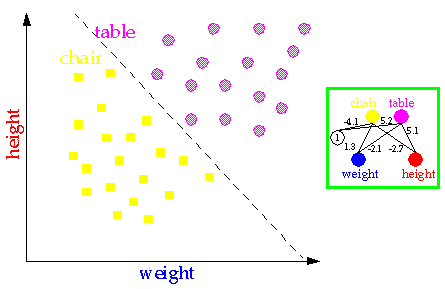More complex classification problems are able to be tackled with more complicated neural architectures; see next figure.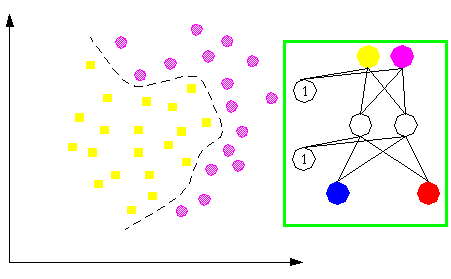In order to learn positive and negative proteins for myristoylation, we used 327 proteins sequenced at the amino acid level that never give rise to myristoylation and 390 positive sequences belonging to 4 sub-groups. More precisely, 234 proteins were supposed to be myristoylated by similarity, 56 proteins were potential candidates for myristoylation and 100 myristoylated proteins had been experimentally proved.

Protein sequences learned by neural networks had 16 amino acids after the N- terminal glycine (see next figure). Neural network input vectors of amino acids were encoded by ``sparse coding''. Each amino acid was transformed into a vector of 20 input neurons with a ``1'' at a particular position and a ``0'' in the others. As 16 amino acids after the glycine site were taken into account, we obtained 320 input neurons. Furthermore, we added the properties of amino acids. With 20 possible binary properties such as charge, size, hydrophobicity for each amino acid, we obtained another vector of 320 input neurons for a total of 640 input neurons.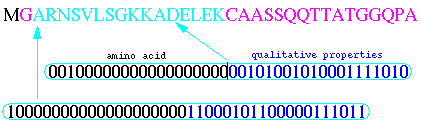The multi-layer perceptron model has been widely used in classification problems. It is characterized by several layers of neurons, as shown in the next figure.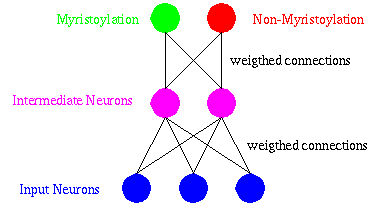DIMLP is a special multi-layer perceptron for which symbolic rules are generated to explain the knowledge embedded within the connections and the activations of neurons. Moreover, the computational complexity of the rule extraction algorithm scales in polynomial time with the dimensionality of the problem, the number of training examples, and the size of the network. Learning is achieved by determining the values of the weights which classify the training examples in the correct classes. Weights are adapted by an optimization algorithm founded on the back-propagation of the gradient.

Recently, the idea that aggregating the opinions of a committee of experts will increase accuracy has been applied in many domains. Note that even in the eighteenth century, the Condorcet Jury Theorem stated that under several conditions an ensemble of predictors will have a higher accuracy than the best of the component predictors. The next figure illustrates an example of an ensemble of three neural networks.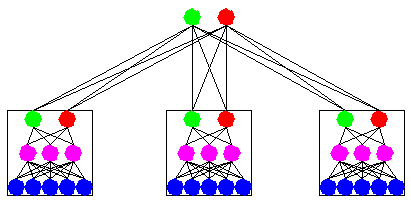To build ensembles of DIMLP networks, we adopted the Bagging method introduced by Breiman. Bagging is based on resampling techniques. Assuming that P is the size of the original training set, bagging generates for each classifier P samples drawn with replacement from the original training set. As a consequence, for each network many of the generated samples may be repeated while others may be left out (see next figure).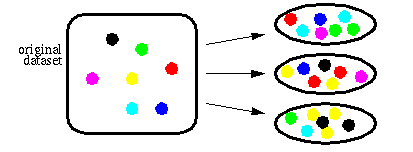The diversity of the training sets contributes to the improvement of the ensemble of neural networks. In practice, preliminary experiments showed that single DIMLP networks were less accurate than ensembles of DIMLP networks for the myristoylation classification problem.

Neural networks are estimated on the proportion of correct responses also denoted as accuracy. Usually, as shown in the next figure a dataset is split into a training set and a testing set. The training set is used to determine the weights of the neural networks, whereas the testing set is used after the training phase to estimate the accuracy.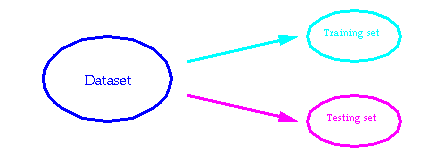We estimated the accuracy of our predictor by leave-one-out. In a leave-one-out trial, the training set is represented by all the data set without a sample, and the testing set has only a sample. After the first run, samples in the training and testing sets are shifted by one position. Nevertheless, with very similar sequences, it is obvious that a leave-one-out procedure will yield better results than with a non-redundant set. Therefore, for each leave-one-out trial all those training sequences which presented more than 30% similarity with the testing sample were removed.

A protein domain is a region within a protein that has been distinguished by a well defined set of properties or characteristics. PROSITE is a database of protein domains developed by the Swiss-Prot group. The PROSITE pattern for myristoylation is defined as:

`G-{EDRKHPFYW}-x(2)-[STAGCN]-{P}.`

The description of the PROSITE pattern is:
• Position 2: uncharged amino acids are allowed. Proline (P), charged and large hydrophobic amino acids are not allowed.
• In positions 3 and 4, most, if not all, amino acids are allowed.
• Position 5: small uncharged amino acids are allowed (A, C, G, N, S and T). Serine (S) is favored.
• Position 6: proline (P) is not allowed.
We defined ensembles of 25 DIMLP networks. The results are illustrated in the next table. Sensitivity is defined as the proportion of positive proteins that tested positive, whereas specificity is the proportion of negative proteins that tested negative. The last row is related to the PROSITE pattern for myristoylation.

SENSITIVITY(%) SPECIFICITY(%)
DIMLP (aa)
86.7
95.4
DIMLP (aa + prop)
93.8
97.9
PROSITE
93.6
77.7

Our neural network ensembles clearly improved specificity. During leave-one-out trials rules were extracted from ensembles of DIMLP networks. It clearly appeared that the most recurrent positions are those specified by the PROSITE pattern. Several properties in agreement with PROSITE favor myristoylation, such as NOT LARGE and NEUTRAL in position 2. NEUTRAL, NOT LARGE and TINY in position 5. Serine (S) in position five is favored. After position six, it is difficult with our current knowledge to give any interpretation. During leave-one-out trials we extracted rulesets with 30.7 rules and 3.7 antecedents per rule, on average. Below we give several examples of rules for myristoylation.
• IF (P_2 = NOT LARGE) AND (P_5 = S) THEN MYR
• IF (P_5 = S) AND (P_6 = POSITIVE) THEN MYR
• IF (P_2 = A) AND (P_5 = NEUTRAL) AND (P_5 = NOT LARGE) THEN MYR
• IF (P_5 = S) AND (P_13 = NEGATIVE) THEN MYR
• IF (P_2 = NEUTRAL) AND (P_4 = LARGE) AND (P_5 = TINY) AND (P_6 = POSITIVE) THEN MYR
• IF (P_2 = N) AND (P_5 = TINY) THEN MYR

Reference:
G. Bologna, C. Yvon, S. Duvaud, A.-L. Veuthey.
N-terminal Myristoylation Predictions by Ensembles of Neural Networks.
Proteomics, In Press.